# Go Math Grade 8 Answer Key Chapter 12 The Pythagorean Theorem

Go Math Grade 8 Answer Key Chapter 12 The Pythagorean Theorem contains 8th Standard Go Math solutions which will make students understand the concepts easily help the students to score well in the exams. This Go Math Grade 8 Answer Key Chapter 12 The Pythagorean Theorem. And in this, each and every question was explained intimately. The answers in this chapter are explained in a simple way that anyone can understand easily.

## Go Math Grade 8 Answer Key Chapter 12 The Pythagorean Theorem

This chapter 12 contains Pythagorean Theorem,Converse of the Pythagorean Theorem, etc. are explained clearly which makes the scholars learn quickly. Go Math Grade 8 Answer Key Chapter 12 Chapter 12 The Pythagorean Theorem. questions are explained in a basic way that students will never feel any difficulty in learning. By this, students can gain good knowledge and this is helpful in finish student’s assignments also.

Chapter 12- Lesson 1:

Chapter 12- Lesson 2:

Chapter 12- Lesson 3:

### Guided Practice – The Pythagorean Theorem – Page No. 378

Question 1.
Find the length of the missing side of the trianglea2 + b2 = c2 → 242 + ? = c2 → ? = c2
The length of the hypotenuse is _____ feet.
_____ feet

Answer: The length of the hypotenuse is 26 feet.

Explanation: According to the Pythagorean Theorem, we shall consider values of a = 24ft, b = 10ft.
Therefore c = √(a2 +b2)
c = √(242 + 102)
= √(576 + 100)
= √676
= 26ft

The Pythagorean Theorem Answer Key Question 2.
Mr. Woo wants to ship a fishing rod that is 42 inches long to his son. He has a box with the dimensions shown.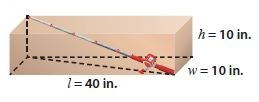a. Find the square of the length of the diagonal across the bottom of the box.
________ inches

Explanation: Here we consider the length of the diagonal across the bottom of the box as d.
Therefore, according to Pythagorean Theorem
W2 + l2 = d2
402 + 102 = d2
1600 + 100 = d2
1700 = d2

Question 2.
b. Find the length from the bottom corner to the opposite top corner to the nearest tenth. Will the fishing rod fit?
________ inches

Explanation: We denote by r, the length from the bottom corner to the opposite top corner. We use our Pythagorean formula to find r.
h2 + s2 = r2
102 + 1700 = r2
100 + 1700 = r2
1800 = r2,    r = √1800 => 42.42 inches

ESSENTIAL QUESTION CHECK-IN

Question 3.
State the Pythagorean Theorem and tell how you can use it to solve problems.

Pythagorean Theorem: In a right triangle, the sum of squares of the legs a and b is equal to the square of the hypotenuse c.
a2 + b2 = c2
We can use it to find the length of a side of a right triangle when the lengths of the other two sides are known.

### 12.1 Independent Practice – The Pythagorean Theorem – Page No. 379

Find the length of the missing side of each triangle. Round your answers to the nearest tenth.

Question 4.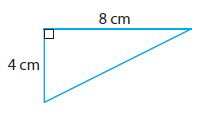________ cm

Explanation: According to the Pythagorean theorem we consider values of a = 4cm, b = 8cm.
c2 = a2 + b2
= 42 + 82
= 16 + 64
c2= 80, c= √80 => 8.944
After rounding to the nearest tenth value c= 8.9cm

Pythagorean Theorem Quiz 8th Grade Math Question 5.________ in.

Explanation: According to the Pythagorean theorem we consider values of b = 8in, c= 14in
c2 = a2 + b2
142 = a2 + 82
196 = a2 + 64
a2 = 196 – 64
a  = √132 => 11.4891
a = 11.5 in

Question 6.
The diagonal of a rectangular big-screen TV screen measures 152 cm. The length measures 132 cm. What is the height of the screen?
________ cm

Explanation: Let’s consider the diagonal of the TV screen as C = 152cm, length as A = 132 cm, and height of the screen as B.As C2 = A2 + B2
1522 = 1322 + B2
23,104 = 17,424 + B2
B2 = 23,104 – 17,424
B = √5680 => 75.365
So the height of the screen B = 75.4cm

Question 7.
Dylan has a square piece of metal that measures 10 inches on each side. He cuts the metal along the diagonal, forming two right triangles. What is the length of the hypotenuse of each right triangle to the nearest tenth of an inch?
________ in.

Explanation: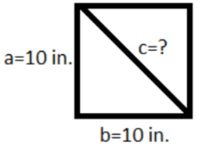Using the Pythagorean Theorem, we have:
a2 + b2 = c2
102 + 102 = c2
100 + 100 = c2
200 = c2
We are told to round the length of the hypotenuse of each right triangle to the nearest tenth of an inch, therefore: c = 14.1in

Question 8.
Represent Real-World Problems A painter has a 24-foot ladder that he is using to paint a house. For safety reasons, the ladder must be placed at least 8 feet from the base of the side of the house. To the nearest tenth of a foot, how high can the ladder safely reach?
________ ft

Explanation: Consider the below diagram. Length of the ladder C = 24ft, placed at a distance from the base B = 8ft, let the safest height be A.By using the Pythagorean Theorem:
C2 = A2 + B2
242 = A2 + 82
576 = A2 + 64
A2 = 576 – 64 => 512
A = √512 => 22.627
After rounding to the nearest tenth, the value of A = 22.6ft

Pythagorean Theorem Unit Test Answer Key Question 9.
What is the longest flagpole (in whole feet) that could be shipped in a box that measures 2 ft by 2 ft by 12 ft?________ ft

Answer: The longest flagpole (in whole feet) that could be shipped in this box is 12 feet.

Explanation:
From the above diagram, we have to find the value of r, which gives us the length longest flagpole that could be shipped in the box.
Where width w = 2ft, height h = 2ft and length l = 12ft.

First find s, the length of the diagonal across the bottom of the box.
w2 + l2 = s2
22 + 122 = s2
4 + 144 = s2
148 = s2
We use our expression for s to find r, since triangles with sides s, r, and h also form a right-angle triangle.
h2 + s2 = r2
22 + 148 = r2
4 + 148 = r2
152 = r2
r = 12.33ft.

Question 10.
Sports American football fields measure 100 yards long between the end zones and are 53 $$\frac{1}{3}$$ yards wide. Is the length of the diagonal across this field more or less than 120 yards? Explain.
____________

Answer: The diagonal across this field is less than 120 yards.

Explanation: From the above details we will get a diagram as shown below.We are given l = 100 and w = 53  =  . If we denote with d the diagonal of the field, using the Pythagorean Theorem, we have:
l2 + w2 = d2
1002 + (160/3)2 = d2
10000 + (25600/9) = d2
9*10000 + 9*(25600/9) = 9* d2
90000 + 25600 = 9 d2
(115600/9) = d2
(340/9) = d2
d = 113.3
Hence the diagonal across this field is less than 120 yards.

Question 11.
Justify Reasoning A tree struck by lightning broke at a point 12 ft above the ground as shown. What was the height of the tree to the nearest tenth of a foot? Explain your reasoning.________ ft

Answer: The total height of the tree was 52.8ft

Explanation: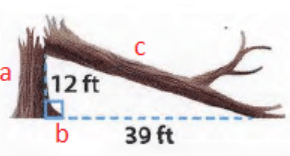By using the Pythagorean Theorem
a2 + b= c2
122 + 392 = c2
144 + 1521 = c2
1665 = c2
We are told to round the length of the hypotenuse to the nearest tenth of a foot, therefore: c = 40.8ft.
Therefore, the total height of the tree was:
height = a+c
height = 12 +40.8
height = 52.8 feet

### FOCUS ON HIGHER ORDER THINKING – The Pythagorean Theorem – Page No. 380

Question 12.
Multistep Main Street and Washington Avenue meet at a right angle. A large park begins at this corner. Joe’s school lies at the opposite corner of the park. Usually Joe walks 1.2 miles along Main Street and then 0.9 miles up Washington Avenue to get to school. Today he walked in a straight path across the park and returned home along the same path. What is the difference in distance between the two round trips? Explain.
________ mi

Answer: Joe walks 1.2 miles less if he follows the straight path across the park.

Explanation: Using the Pythagorean Theorem, we find the distance from his home to school following the straight path across the park:
a2 + b= c2
1.22 + 0.92 = c2
1.44 + 0.81 = c2
2.25 = c2
1.5 = c
Therefore, the distance of Joe’s round trip following the path across the park is 3 miles (dhome-school + dschool-home = 1.5 + 1.5). Usually, when he walks along Main Street and Washington Avenue, the distance of his round trip is 4.2 miles (dhome-school + dschool-home = (1.2 + 0.9) + (0.9+1.2)). As we can see, Joe walks 1.2 miles less if he follows the straight path across the park.

Question 13.
Analyze Relationships An isosceles right triangle is a right triangle with congruent legs. If the length of each leg is represented by x, what algebraic expression can be used to represent the length of the hypotenuse? Explain your reasoning.

Explanation: From the Pythagorean Theorem, we know that if a and b are legs and c is the hypotenuse, then a2 + b= c2. In our case, the length of each leg is represented by x, therefore we have:
a2 + b= c2
x2 + x2 = c2
2x2 = c2
c = x√ 2

Pythagorean Theorem 8th Grade Math Question 14.
Persevere in Problem-Solving A square hamburger is centered on a circular bun. Both the bun and the burger have an area of 16 square inches.a. How far, to the nearest hundredth of an inch, does each corner of the burger stick out from the bun? Explain.
________ in

Answer: Each corner of the burger sticks out 0.57 inches from the bun.

Explanation: First, we need to find the radius r of the circular bun. We know that its area A is 16 square inches, therefore:A = πr2
16 = 3.14*r2
r2 = (16/3.14)
r = 2.26

Then, we need to find the side s of the square hamburger. We know that its area A is 16 square inches, therefore:
A = s2
16 = s2
s = 4
Using the Pythagorean Theorem, we have to find the diagonal d of the square hamburger:
s2 + s2 = d2
42 + 42 = d2
16 + 16 = d2
32 = d2
d = 5.66
To find how far each corner of the burger sticks out from the bun, we denote this length by a and we get:
a = (d/2) – r => (5.66/2) – 2.26
a = 0.57.
Therefore, Each corner of the burger sticks out 0.57 inches from the bun.

Question 14.
b. How far does each bun stick out from the center of each side of the burger?
________ in

Answer: Each bun sticks out 0.26 inches from the center of each side of the burger.

Explanation:We found that r = 2.26 and s = 4. To find how far each bun sticks out from the center of each side of the burger, we denote this length by b and we get:
b = r – (s/2) = 2.26 – (4/2)
b = 0.26 inches.

Question 14.
c. Are the distances in part a and part b equal? If not, which sticks out more, the burger or the bun? Explain.
The distances a and b are not equal. From the calculations, we found that the burger sticks out more than the bun.

### Guided Practice – Converse of the Pythagorean Theorem – Page No. 384

Question 1.
Lashandra used grid paper to construct the triangle shown.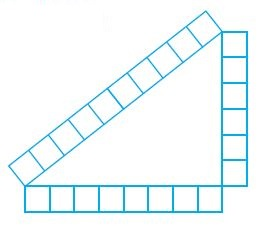a. What are the lengths of the sides of Lashandra’s triangle?
_______ units, _______ units, _______ units,

Answer: The length of Lashandra’s triangle is 8 units, 6 units, 10 units.

Question 1.
b. Use the converse of the Pythagorean Theorem to determine whether the triangle is a right triangle.The triangle that Lashandra constructed is/is not a right triangle.
_______ a right triangle

Answer: Lashandra’s triangle is right angled triangle as it satisfied the Pythagorean theorem

Explanation:
Verifying with Pythagorean formula a2 + b= c2
82 + 62 = 102
64 + 36 =100
100 = 100.

Understand The Pythagorean Theorem Answer Key Question 2.
A triangle has side lengths of 9 cm, 12 cm, and 16 cm. Tell whether the triangle is a right triangle.
Let a = _____, b = _____, and c = ______.By the converse of the Pythagorean Theorem, the triangle is/is not a right triangle.
_______ a right triangle

Answer: The given triangle is not a right-angled triangle

Explanation: Verifying with Pythagorean formula a2 + b= c2
92 + 122 = 162
81 + 144 = 256
225 ≠ 256.
Hence given dimensions are not from the right-angled triangle.

Question 3.
The marketing team at a new electronics company is designing a logo that contains a circle and a triangle. On one design, the triangle’s side lengths are 2.5 in., 6 in., and 6.5 in. Is the triangle a right triangle? Explain.
_______

Answer: It is a right-angled triangle.

Explanation: Let a = 2.5, b = 6 and c= 6.5
Verifying with Pythagorean formula a2 + b= c2
2.52 + 62 = 6.52
6.25 + 36 = 42.25
42.25 = 42.25.
Hence it is a right-angled triangle.

ESSENTIAL QUESTION CHECK-IN

Question 4.
How can you use the converse of the Pythagorean Theorem to tell if a triangle is a right triangle?

Answer: Knowing the side lengths, we substitute them in the formula a2 + b= c2, where c contains the biggest value. If the equation holds true, then the given triangle is a right triangle. Otherwise, it is not a right triangle.

### 12.2 Independent Practice – Converse of the Pythagorean Theorem – Page No. 385

Tell whether each triangle with the given side lengths is a right triangle.

Question 5.
11 cm, 60 cm, 61 cm
______________

Answer: Since 112 + 602 = 612, the triangle is a right-angled triangle.

Explanation: Let a = 11, b = 60 and c= 61
Using the converse of the Pythagorean Theorem a2 + b= c2
112 + 602 = 612
121 + 3600 = 3721
3721 = 3721.
Since 112 + 602 = 612, the triangle is a right-angled triangle.

Question 6.
5 ft, 12 ft, 15 ft
______________

Answer: Since 52 + 122 ≠ 152, the triangle is not a right-angled triangle.

Explanation: Let a = 5, b = 12 and c= 15
Using the converse of the Pythagorean Theorem a2 + b= c2
52 + 122 = 152
25 + 144 = 225
169 ≠ 225.
Since 52 + 122 ≠ 152, the triangle is not a right-angled triangle.

Pythagorean Theorem Quick Check Answer Key Question 7.
9 in., 15 in., 17 in.
______________

Answer: Since 92 + 152 ≠ 172, the triangle is not a right-angled triangle.

Explanation: Let a = 9, b = 15 and c= 17
Using the converse of the Pythagorean Theorem a2 + b= c2
92 + 152 = 172
81 + 225 = 225
306 ≠ 225.
Since 92 + 152 ≠ 172, the triangle is not a right-angled triangle.

Question 8.
15 m, 36 m, 39 m
______________

Answer: Since 152 + 362 = 392, the triangle is a right-angled triangle.

Explanation: Let a = 15, b = 36 and c= 39
Using the converse of the Pythagorean Theorem a2 + b= c2
152 + 362 = 392
225 + 1296 = 1521
1521 = 1521.
Since 152 + 362 = 392, the triangle is a right-angled triangle.

Question 9.
20 mm, 30 mm, 40 mm
______________

Answer: Since 202 + 302 ≠ 402, the triangle is not a right-angled triangle.

Explanation: Let a = 20, b = 30 and c= 40
Using the converse of the Pythagorean Theorem a2 + b= c2
202 + 302 = 402
400 + 900 = 1600
1300 ≠ 1600.
Since 202 + 302 ≠ 402, the triangle is not a right-angled triangle.

Question 10.
20 cm, 48 cm, 52 cm
______________

Answer: Since 202 + 482 = 522, the triangle is a right-angled triangle.

Explanation: Let a = 20, b = 48 and c= 52
Using the converse of the Pythagorean Theorem a2 + b= c2
202 + 482 = 522
400 + 2304 = 2704
2704 = 2704.
Since 202 + 482 = 522, the triangle is a right-angled triangle.

Question 11.
18.5 ft, 6 ft, 17.5 ft
______________

Answer: Since 62 + 17.52 = 18.52, the triangle is a right-angled triangle.

Explanation: Let a = 6, b = 17.5 and c= 18.5
Using the converse of the Pythagorean Theorem a2 + b= c2
62 + 17.52 = 18.52
36 + 306.25 = 342.25
342.5 = 342.25.
Since 62 + 17.52 = 18.52, the triangle is a right-angled triangle.

Question 12.
2 mi, 1.5 mi, 2.5 mi
______________

Answer: Since 22 + 1.52 = 2.52, the triangle is a right-angled triangle.

Explanation: Let a = 2, b = 1.5 and c= 2.5
Using the converse of the Pythagorean Theorem a2 + b= c2
22 + 1.52 = 2.52
4 + 2.25 = 6.25
6.25 = 6.25.
Since  22 + 1.52 = 2.52, the triangle is a right-angled triangle.

Question 13.
35 in., 45 in., 55 in.
______________

Answer: Since 352 + 452 ≠ 552, the triangle is not a right-angled triangle.

Explanation: Let a = 35, b = 45 and c= 55
Using the converse of the Pythagorean Theorem a2 + b= c2
352 + 452 = 552
1225 + 2025 = 3025
3250 ≠ 3025.
Since 352 + 452 ≠ 552, the triangle is not a right-angled triangle.

Question 14.
25 cm, 14 cm, 23 cm
______________

Answer: Since  142 + 232 ≠ 252, the triangle is not a right-angled triangle.

Explanation: Let a = 14, b = 23 and c= 25 (longest side)
Using the converse of the Pythagorean Theorem a2 + b= c2
142 + 232 = 252
196 + 529 = 625
725 ≠ 625.
Since  142 + 232 ≠252, the triangle is not a right-angled triangle.

Question 15.
The emblem on a college banner consists of the face of a tiger inside a triangle. The lengths of the sides of the triangle are 13 cm, 14 cm, and 15 cm. Is the triangle a right triangle? Explain.
________

Answer: Since  132 + 142 ≠ 152, the triangle is not a right-angled triangle.

Explanation: Let a = 13, b = 14 and c= 15
Using the converse of the Pythagorean Theorem a2 + b= c2
132 + 142 = 152
169 + 196 = 225
365 ≠ 225.
Since  132 + 142 ≠ 152, the triangle is not a right-angled triangle.

Question 16.
Kerry has a large triangular piece of fabric that she wants to attach to the ceiling in her bedroom. The sides of the piece of fabric measure 4.8 ft, 6.4 ft, and 8 ft. Is the fabric in the shape of a right triangle? Explain.
________

Answer: The triangular piece of fabric that Kerry has is in the shape of a right angle since it follows the Pythagorean theorem.

Explanation: Let a = 4.8, b = 6.4 and c= 8
Using the converse of the Pythagorean Theorem a2 + b= c2
4.82 + 6.42 = 82
23.04 + 40.96 = 64
64 = 64.
Since 4.82 + 6.42 = 82, the triangle is a right-angled triangle.

Question 17.
A mosaic consists of triangular tiles. The smallest tiles have side lengths 6 cm, 10 cm, and 12 cm. Are these tiles in the shape of right triangles? Explain.
________

Answer: Since 62 + 102 ≠ 122, by the converse of the Pythagorean Theorem, we say that the tiles are not in the shape of a right-angled triangle.

Explanation: Let a = 6, b = 10 and c= 12
Using the converse of the Pythagorean Theorem a2 + b= c2
62 + 102 = 122
36 + 100 = 144
136 ≠ 144.
Since 62 + 102 ≠ 122, by the converse of the Pythagorean Theorem, we say that the tiles are not in the shape of a right-angled triangle.

The Pythagorean Theorem and Maps Answer Key Question 18.
History In ancient Egypt, surveyors made right angles by stretching a rope with evenly spaced knots as shown. Explain why the rope forms a right angle.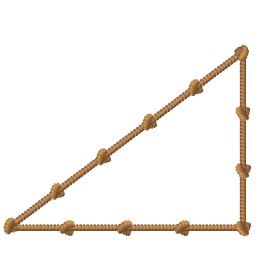Answer: The rope has formed a right-angled triangle because the length of its sides follows the Pythagorean Theorem.

Explanation: The knots are evenly placed at equal distances
The lengths in terms of knots are a=4 knots, b = 3knots, c = 5 knots
Therefore a2 + b= c2
42 + 3= 52
16+9 = 25
25 = 25.
Hence rope has formed a right-angled triangle because the length of its sides follows Pythagorean Theorem.

### Converse of the Pythagorean Theorem – Page No. 386

Question 19.
Justify Reasoning Yoshi has two identical triangular boards as shown. Can he use these two boards to form a rectangle? Explain.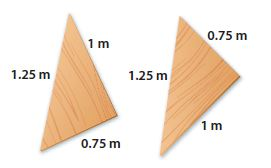Answer: Since it was proved that both can form a right-angled triangle, we can form a rectangle by joining them.

Explanation: Given both triangles are identical, if both are right-angled triangles then we can surely join to form a rectangle.
Let’s consider a = 0.75, b= 1 and c=1.25.
By using converse Pythagorean Theorem a2 + b= c2
0.752 + 12 = 1.252
0.5625 + 1 = 1.5625
1.5625 = 1.5625.
Since it was proved that both can form a right-angled triangle, we can form a rectangle by joining them.

12.2 Pythagorean Theorem Answer Key Question 20.
Critique Reasoning Shoshanna says that a triangle with side lengths 17 m, 8 m, and 15 m is not a right triangle because 172 + 82 = 353, 152 = 225, and 353 ≠ 225. Is she correct? Explain
_______

Answer: She is not right, A triangle with sides 15, 8, and 17 is a right-angled triangle.

Explanation: Lets consider a =15, b= 8 and c = 17 (which is long side)
We will verify by using converse Pythagorean Theorem a2 + b= c2
152 + 82 = 172
225 + 64 = 289
289 = 289.
Since the given dimensions satisfied the Pythagorean Theorem, we can say it is a right-angled triangle. In the given above statement what Shoshanna did was c2 + b2 = a2, which is not the correct definition of the Pythagorean Theorem.

FOCUS ON HIGHER ORDER THINKING

Question 21.
Make a Conjecture Diondre says that he can take any right triangle and make a new right triangle just by doubling the side lengths. Is Diondre’s conjecture true? Test his conjecture using three different right triangles.
_______

Answer: Yes, Diondre’s conjecture is true. By doubling the sides of a right triangle would create a new right triangle.

Explanation: Given a right triangle, the Pythagorean Theorem holds. Therefore, a2 + b= c2
If we double the side lengths of that triangle, we get:
(2a)2 + (2b)= (2c)2
4a2 + 4b2 = 4c2
4(a2 + b2) = 4c2
a2 + b= c2
As we can see doubling the sides of a right triangle would create a new right triangle.We can test that by using three different right triangles.

The triangle with sides a = 6, b = 8, and c = 10 is a right triangle. We double its sides and check if the new triangle is a right triangle. After doubling the value of a = 12, b = 16 and c = 20.
122 + 162 = 202
144 + 256 = 400
400 = 400
Hence proved!
Since 122 + 162 = 202, the new triangle is a right triangle by the converse of the Pythagorean Theorem.

The triangle with sides a = 3, b = 4 and c = 5 is a right triangle. We double its sides and check if the new triangle is a right triangle. After doubling the value of a = 6, b = 8 and c = 10.
62 + 82 = 102
36 + 64 = 100
100 = 100
Hence proved!
Since 62 + 82 = 102, the new triangle is a right triangle by the converse of the Pythagorean Theorem.

The triangle with sides a = 12, b = 16 and c = 20 is a right triangle. We double its sides and check if the new triangle is a right triangle. After doubling the value of a = 24, b = 32 and c = 40.
242 + 322 = 402
576 + 1024 = 1600
1600 = 1600
Hence proved!
Since 242 + 322 = 402, the new triangle is a right triangle by the converse of the Pythagorean Theorem.

Question 22.
Draw Conclusions A diagonal of a parallelogram measures 37 inches. The sides measure 35 inches and 1 foot. Is the parallelogram a rectangle? Explain your reasoning.
_______

Answer: Since 122 + 352 = 372, the triangle is the right triangle. Therefore, the given parallelogram is a rectangle.

Explanation: A rectangle is a parallelogram where the interior angles are right angles. To prove if the given parallelogram is a rectangle, we need to prove that the triangle formed by the diagonal of the parallelogram and two sides of it, is a right triangle. Converting all the values into inches, we have a = 12, b = 35 and c = 37. Using the converse of the Pythagorean Theorem, we have:
a2 + b= c2
122 + 352 = 372
144 + 1225 = 1369
1369 = 1369.
Since 122 + 352 = 372, the triangle is the right triangle. Therefore, the given parallelogram is a rectangle.

Question 23.
Represent Real-World Problems A soccer coach is marking the lines for a soccer field on a large recreation field. The dimensions of the field are to be 90 yards by 48 yards. Describe a procedure she could use to confirm that the sides of the field meet at right angles.

Answer: To confirm that the sides of the field meet at right angles, she could measure the diagonal of the field and use the converse of the Pythagorean Theorem. If a2 + b= c2 (where a = 90, b = 48, and c is the length of the diagonal), then the triangle is the right triangle. This method can be used for every corner to decide if they form right angles or not.

### Guided Practice – Distance Between Two Points – Page No. 390

Question 1.
Approximate the length of the hypotenuse of the right triangle to the nearest tenth using a calculator._______ units

Answer: The length of the hypotenuse of the right triangle to the nearest tenth is 5.8 units.

Explanation: From the above figure let’s take
Length of the vertical leg = 3 units
Length of the horizontal leg = 5 units
let length of the hypotenuse = c
By using Pythagorean Theorem a2 + b= c2
c2 = 32 + 52
c2 = 9 +25
c = √34 => 5.830.
Therefore Length of the hypotenuse of the right triangle to the nearest tenth is 5.8 units.

Question 2.
Find the distance between the points (3, 7) and (15, 12) on the coordinate plane.
_______ units

Answer: The distance between points on the coordinate plane is 13

Explanation: So (x1, y1) = (3,7) and  (x2, y2) = (15, 12)
distance formula d = √( x2 – x1)2 + √( y2 – y1)2
d = √(15 -3)2 + √(12 – 7)2
d = √122 + 52
d = √144 + 25
d = √169 => 13
Therefore distance between points on the coordinate plane is 13.

Lesson 2 Pythagorean Theorem Answer Key Question 3.
A plane leaves an airport and flies due north. Two minutes later, a second plane leaves the same airport flying due east. The flight plan shows the coordinates of the two planes 10 minutes later. The distances in the graph are measured in miles. Use the Pythagorean Theorem to find the distance shown between the two planes._______ miles

Answer: The distance between the two planes is 103.6 miles.

Explanation:
Length of the vertical dv = √(80 -1)2 + √(1-1)2
= √792 => 79.
Length of the horizontal dh = √(68 -1)2 + √(1-1)2
= √672 => 67.
Distance between the two planes D = √(792 + 672)
= √(6241+4489) => √10730
= 103.5857 => 103.6 miles.

ESSENTIAL QUESTION CHECK-IN

Question 4.
Describe two ways to find the distance between two points on a coordinate plane.

Explanation: We can draw a right triangle whose hypotenuse is the segment connecting the two points and then use the Pythagorean Theorem to find the length of that segment. We can also the Distance formula to find the length of that segment.

For example, plot three points; (1,2), (20,2) and (20,12)

Using the Pythagorean Theorem:

The length of the horizontal leg is the absolute value of the difference between the x-coordinates of the points (1,2) and (20,2).
|1 – 20| = 19
The length of the horizontal leg is 19.

The length of the vertical leg is the absolute value of the difference between the y-coordinates of the points (20,2) and (20,12).
|2 – 12| = 10
The length of the vertical leg is 10.

Let a = 19, b = 10 and let c represent the hypotenuse. Find c.
a2 + b= c2
192 + 10= c2
361 + 100 = c2
461 = c2
distance is 21.5 = c

Using the Distance formula:
d= √( x2 – x1)2 + √( y2 – y1)2
The length of the horizontal leg is between (1,2) and (20,2).
d= √( x2 – x1)2 + √( y2 – y1)2
=  √(20 -1)2 + √(2-2)2
= √(19)2 + √(0)2
= √361 => 19
The length of the vertical leg is between (20,2) and (20,12).
d= √( x2 – x1)2 + √( y2 – y1)2
=  √(20 -20)2 + √(12-2)2
= √(0)2 +√(10)2
= √100 => 10
The length of the diagonal leg is between (1,2) and (20,12).
d= √( x2 – x1)2 + √( y2 – y1)2
=  √(20 -1)2 + √(12-2)2
= √(19)2 + √(10)2
= √(361+100) => √461 = 21.5

### 12.3 Independent Practice – Distance Between Two Points – Page No. 391

Question 5.
A metal worker traced a triangular piece of sheet metal on a coordinate plane, as shown. The units represent inches. What is the length of the longest side of the metal triangle? Approximate the length to the nearest tenth of an inch using a calculator. Check that your answer is reasonable.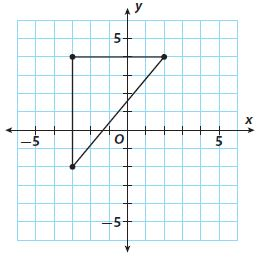_______ in.

Answer: The length of the longest side of the metal triangle to the nearest tenth is 7.8 units.

Explanation: From the above figure let’s take
Length of the vertical leg = 6 units
Length of the horizontal leg = 5 units
let length of the hypotenuse = c
By using Pythagorean Theorem a2 + b= c2
c2 = 62 + 52
c2 = 36 +25
c = √61 => 7.8
Therefore Length of the longest side of the metal triangle to the nearest tenth is 7.8 units.

Question 6.
When a coordinate grid is superimposed on a map of Harrisburg, the high school is located at (17, 21) and the town park is located at (28, 13). If each unit represents 1 mile, how many miles apart are the high school and the town park? Round your answer to the nearest tenth.
_______ miles

Answer: The high school and the town park are 13.6 miles apart.

Explanation: The coordinates of the high school are said to be (17,21), whereas the coordinates of the park are (28,13). In a coordinate plane, the distance d between the points (17,21) and (28,13) is:

d= √( x2 – x1)2 + √( y2 – y1)2
=  √(28 -17)2 + √(13-21)2
= √(11)2 + √(-8)2
= √(121+64) => √185 = 13.6014

Rounding the answer to the nearest tenth:
d = 13.6.
Taking into consideration that each unit represents 1 mile, the high school and town park are 13.6 miles apart.

Question 7.
The coordinates of the vertices of a rectangle are given by R(- 3, – 4), E(- 3, 4), C (4, 4), and T (4, – 4). Plot these points on the coordinate plane at the right and connect them to draw the rectangle. Then connect points E and T to form diagonal $$\overline { ET }$$.
a. Use the Pythagorean Theorem to find the exact length of $$\overline { ET }$$.Explanation:
Taking into consideration the triangle TRE, the length of the vertical leg (ER) is 8 units. The length of the horizontal leg (RT) is 7 units. Let a = 8 and b =7. Let c represent the length of the hypotenuse, the diagonal ET. We use the Pythagorean Theorem to find c.
a2 + b= c2
c2 = 82 + 72
c2 = 64 +49
c = √113 => 10,63.
The diagonal ET is about 10.63 units long.

Question 7.
b. How can you use the Distance Formula to find the length of $$\overline { ET }$$ ? Show that the Distance Formula gives the same answer.

Answer: The diagonal ET is about 10.63 units long. As we can see the answer is the same as the one we found using the Pythagorean Theorem.

Explanation: Using the distance formula, in a coordinate plane, the distance d between the points E(-3,4) and T(4, -4) is:
d= √( x2 – x1)2 + √( y2 – y1)2
=  √(4 – (-3))2 + √(- 4 – 4)2
= √(7)2 + √(-8)2
= √(49+64) => √113 = 10.63.
The diagonal ET is about 10.63 units long. As we can see the answer is the same as the one we found using the Pythagorean Theorem.

Grade 8 Lesson 3 Distance in the Coordinate Plane Answer Key Question 8.
Multistep The locations of three ships are represented on a coordinate grid by the following points: P(-2, 5), Q(-7, -5), and R(2, -3). Which ships are farthest apart?

Answer: Ships P and Q are farthest apart

Explanation: Distance Formula: In a coordinate plane, the distance d between two points (x1,y1) and (x2,y2) is: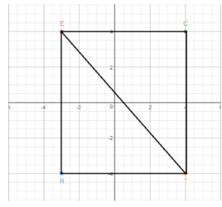d= √( x2 – x1)2 + √( y2 – y1)2
The distance d1 between the two points P(-2,5) and Q(-7,-5) is:
d1 = √( xQ – xP)2 + √( yQ – yP)2
= √(-7 – (-2))2 + √(- 5 – 5)2
= √(-5)2 + √(-10)2
= √(25+100) => √125 = 11.18

The distance d2 between the two points Q(-7,-5) and R(2,-3) is:
d3 = √( xR – xQ)2 + √( yR – yQ)2
= √(2 – (-7))2 + √(- 3 – 5)2
= √(9)2 + √(2)2
= √(81+4) => √85 = 9.22

The distance d3 between the two points P(-2,5) and R(2,-3) is:
d3 = √( xR – xP)2 + √( yR – yP)2
= √(2 – (-2))2 + √(- 3 – 5)2
= √(4)2 + √(-8)2
= √(16+64) => √80 = 8.94.
As we can see, the greatest distance is d1 11.8, which means that ships P and Q are farthest apart.

### Distance Between Two Points – Page No. 392

Question 9.
Make a Conjecture Find as many points as you can that are 5 units from the origin. Make a conjecture about the shape formed if all the points 5 units from the origin were connected.

Explanation: Some of the points that are 5 units away from the origin are: (0,5), (3,4), (4,3),(5,0),(4,-3),(3,-4),(0,-5),(-3,-4),(-4,-3),(-5,0),(-4,3),(-3,4) etc If all the points 5 units away from the origin are connected, a circle would be formed.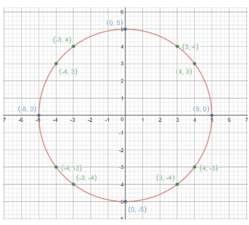Question 10.
Justify Reasoning The graph shows the location of a motion detector that has a maximum range of 34 feet. A peacock at point P displays its tail feathers. Will the motion detector sense this motion? Explain.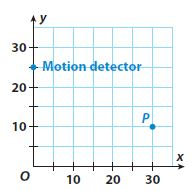Answer: Considering each unit represents 1 foot, the motion detector, and peacock are 33.5 feet apart. Since the motion detector has a maximum range of 34 feet, it means that it will sense the motion of the peacock’s feathers.

Explanation: The coordinates of the motion detector are said to be (0,25), whereas the coordinates of the peacock are (30,10). In a coordinate plane, the distance d between the points (0,25) and (30,10) is:
d = √( x2 – x1)2 + √( y2 – y1)2
= √(30 – 0)2 + √(10 – 25)2
= √(30)2 + √(-15)2
= √(900+225) => √1125.
Rounding answer to the nearest tenth:
d = 33.5 feet.
Considering each unit represents 1 foot, the motion detector and peacock are 33.5 feet apart. Since the motion detector has a maximum range of 34 feet, it means that it will sense the motion of the peacock’s feathers.

FOCUS ON HIGHER ORDER THINKING

Question 11.
Persevere in Problem-Solving One leg of an isosceles right triangle has endpoints (1, 1) and (6, 1). The other leg passes through the point (6, 2). Draw the triangle on the coordinate plane. Then show how you can use the Distance Formula to find the length of the hypotenuse. Round your answer to the nearest tenth.Explanation:One leg of an isosceles right triangle has endpoints (1,1) and (6,1), which means that the leg is 5 units long. Since the triangle is isosceles, the other leg should be 5 units long too, therefore the endpoints of the second leg that passes through the point (6,2) are (6,1) and (6,6).
In the coordinate plane, the length of the hypotenuse is the distance d between the points (1,1) and (6,6).
d = √( x2 – x1)2 + √( y2 – y1)2
= √(6 – 1)2 + √(6 – 1)2
= √(5)2 + √(5)2
= √(25+25) => √50.
d = 7.1.
The hypotenuse is around 7.1 units long.

Question 12.
Represent Real-World Problems The figure shows a representation of a football field. The units represent yards. A sports analyst marks the locations of the football from where it was thrown (point A) and where it was caught (point B). Explain how you can use the Pythagorean Theorem to find the distance the ball was thrown. Then find the distance._______ yards

Answer: The distance between point A and B is 37 yards

Explanation: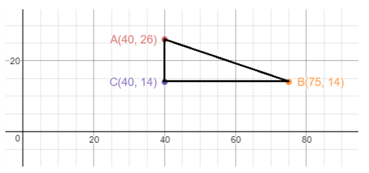To find the distance between points A and B, we draw segment AB and label its length d. Then we draw vertical segment AC and Horizontal segment CB. We label the lengths of these segments a and b. triangle ACB is a right triangle with hypotenuse AB.
Since AC is vertical segment, its length, a, is the difference between its y-coordinates. Therefore, a = 26 – 14 = 12 units.
Since CB is horizontal segment, its length b is the difference between its x-coordinates. Therefore, b = 75 – 40 = 35units.
We use the Pythagorean Theorem to find d, the length of segment AB.
d2 = a2 + b2
d2 = 122 + 352
d2 = 144 + 1225
d2 = 1369 => d = √1369 => 37
The distance between points A and B is 37 yards

### Ready to Go On? – Model Quiz – Page No. 393

12.1 The Pythagorean Theorem

Find the length of the missing side.

Question 1.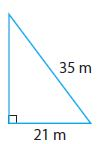________ meters

Answer: Length of missing side is 28m

Explanation: Lets consider value of a = 21 and c = 35.
Using Pythagorean Theorem a2 + b= c2
212 + b2 = 352
441 + b2 = 1225
b2= 784 => b = √784 = 28.
Therefore length of missing side is 28m.

Question 2.________ ft

Answer: Length of missing side is 34ft

Explanation: Let’s consider value of a = 16 and b = 30.
Using Pythagorean Theorem a2 + b= c2
162 + 302 = c2
256 + 900 = c2
c2= 1156 => c = √1156 = 34.
Therefore length of missing side is 34ft.

12.2 Converse of the Pythagorean Theorem

Tell whether each triangle with the given side lengths is a right triangle.

Question 3.
11, 60, 61
____________

Answer: Since 112 + 602 = 612, by the converse of the Pythagorean Theorem, we say that the given sides are in the shape of a right-angled triangle.

Explanation: Let a = 11, b = 60 and c= 61
Using the converse of the Pythagorean Theorem a2 + b= c2
112 + 602 = 612
121 + 3600 = 3721
3721 = 3721
Since 112 + 602 = 612, by the converse of the Pythagorean Theorem, we say that the given sides are in the shape of a right-angled triangle.
Question 4.
9, 37, 40
____________

Answer: Since  92 + 372 ≠ 402, by the converse of the Pythagorean Theorem, we say that the given sides are not in the shape of a right-angled triangle.

Explanation: Let a = 9, b = 37 and c= 40
Using the converse of the Pythagorean Theorem a2 + b= c2
92 + 372 = 402
81 + 1369 = 1600
1450 ≠ 3721.
Since  92 + 372 ≠ 402, by the converse of the Pythagorean Theorem, we say that the given sides are not in the shape of a right-angled triangle.

Pythagorean Theorem Test Grade 8 Question 5.
15, 35, 38
____________

Answer: Since 152 + 352 ≠ 382, by the converse of the Pythagorean Theorem, we say that the given sides are not in the shape of a right-angled triangle.

Explanation: Let a = 15, b = 35 and c= 38
Using the converse of the Pythagorean Theorem a2 + b= c2
152 + 352 = 382
225 + 1225 = 1444
1450 ≠ 1444
Since 152 + 352 ≠ 382, by the converse of the Pythagorean Theorem, we say that the given sides are not in the shape of a right-angled triangle.

Question 6.
28, 45, 53
____________

Answer: Since 282 + 452 = 532, by the converse of the Pythagorean Theorem, we say that the given sides are in the shape of right-angled triangle.

Explanation: Let a = 28, b = 45 and c= 53
Using the converse of the Pythagorean Theorem a2 + b= c2
282 + 452 = 532
784 + 2025 = 2809
2809 = 2809
Since 282 + 452 = 532, by the converse of the Pythagorean Theorem, we say that the given sides are in the shape of right-angled triangle.
Question 7.
Keelie has a triangular-shaped card. The lengths of its sides are 4.5 cm, 6 cm, and 7.5 cm. Is the card a right triangle?
____________

Answer: Since 4.52 + 62 = 7.52, by the converse of the Pythagorean Theorem, we say that the given sides are in the shape of right-angled triangle.

Explanation: Let a = 4.5, b = 6 and c= 7.5
Using the converse of the Pythagorean Theorem a2 + b= c2
4.52 + 62 = 7.52
20.25 + 36 = 56.25
56.25= 56.25
Since 4.52 + 62 = 7.52, by the converse of the Pythagorean Theorem, we say that the given sides are in the shape of right-angled triangle.

12.3 Distance Between Two Points

Find the distance between the given points. Round to the nearest tenth.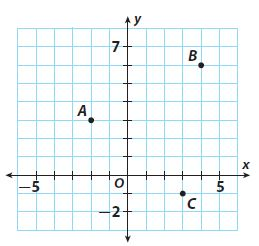Question 8.
A and B
________ units

Answer: The distance between A and B is 6.7 units

Explanation: A= (-2,3) and B= (4,6)

Distance between A and B is d = √( x2 – x1)2 + √( y2 – y1)2
= √(4 – (-2)2 + √(6 – 3)2
= √(6)2 + √(3)2
= √(36+9) => √45 = 6.7 units

Question 9.
B and C
________ units

Answer: The distance between B and C is 7.07 units

Explanation: B= (4,6) and C= (3,1)

Distance between B and C is d = √( x2 – x1)2 + √( y2 – y1)2
= √(4 – 3)2 + √(6 – (-1))2
= √(1)2 + √(7)2
= √(1+49) => √50 = 7.07 units

Question 10.
A and C
________ units

Answer: The distance between A and C is 6.403 units

Explanation: A= (-2,3) and C= (3, -1)

Distance between A and C is d = √( x2 – x1)2 + √( y2 – y1)2
= √(3 – (-2)2 + √(-1 – 3)2
= √(5)2 + √(-4)2
= √(25+16) => √41 = 6.403 units

ESSENTIAL QUESTION

Question 11.
How can you use the Pythagorean Theorem to solve real-world problems?

Answer: We can use the Pythagorean Theorem to find the length of a side of a right triangle when we know the lengths of the other two sides. This application is usually used in architecture or other physical construction projects. For example, it can be used to find the length of a ladder, if we know the height of the wall and distance on the ground from the wall of the ladder.

### Selected Response – Mixed Review – Page No. 394

Question 1.
What is the missing length of the side?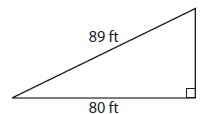A. 9 ft
B. 30 ft
C. 39 ft
D. 120 ft

Explanation:
Given a= 80 ft
b= ?
c= 89 ft
As a2+b2=c 2
802+b2= 892
6,400+b2= 7,921
b2= 7,921-6,400
b= √1,521
b= 39 ft.

Pythagorean Theorem Test Grade 8 Pdf Question 2.
Which relation does not represent a function?
Options:
A. (0, 8), (3, 8), (1, 6)
B. (4, 2), (6, 1), (8, 9)
C. (1, 20), (2, 23), (9, 26)
D. (0, 3), (2, 3), (2, 0)

Explanation: The value of X is the same for 2 points and 2 values of Y [(2, 3), (2, 0)]. The value of X is repeated for a function to exist, no two points can have the same X coordinates.

Question 3.
Two sides of a right triangle have lengths of 72 cm and 97 cm. The third side is not the hypotenuse. How long is the third side?
Options:
A. 25 cm
B. 45 cm
C. 65 cm
D. 121 cm

Explanation:
Given a= 72 cm
b= ?
c= 97 cm
As a2+b2=c 2
722+b2= 972
5,184+b2= 9,409
b2= 9,409-5,184
b= √4,225
b= 65 cm.

Question 4.
To the nearest tenth, what is the distance between point F and point G?Options:
A. 4.5 units
B. 5.0 units
C. 7.3 units
D. 20 units

Explanation:
Given F= (-1,6) =(x1,y1).
G= (3,4) = (x2,y2).
The difference between F&G points is
d= √(x2-x1)2 + (y2-y1)2
=  √(3 – (-1))2 + (4 – 6)2
= √(4)2 + (-2)2
= √16+4
= √20
= 4.471
= 4.5 units.

Question 5.
A flagpole is 53 feet tall. A rope is tied to the top of the flagpole and secured to the ground 28 feet from the base of the flagpole. What is the length of the rope?
Options:
A. 25 feet
B. 45 feet
C. 53 feet
D. 60 feet

Explanation: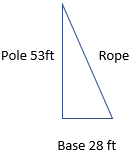By Pythagorean theorem
a2+b2=c 2
532+282= C2
2,809+784= C2
C2 = 9,409-5,184
C2 = 3,593
C= √3,593
C= 59.94 feet
=60 feet.

Question 6.
Which set of lengths are not the side lengths of a right triangle?
Options:
A. 36, 77, 85
B. 20, 99, 101
C. 27, 120, 123
D. 24, 33, 42

Explanation:
Check if side lengths in option A form a right triangle.
Let a= 36, b= 77, c= 85
By Pythagorean theorem
a2+b2=c 2
362+772= 852
1,296+ 5,929= 7,225
7,225= 7,225
As 362+772= 852 the triangle is a right triangle.

Check if side lengths in option B form a right triangle.
Let a= 20, b= 99, c= 101
By Pythagorean theorem
a2+b2=c 2
202+992= 1012
400+ 9,801= 10,201
10,201= 10,201
As 202+992= 1012 the triangle is a right triangle.

Check if side lengths in option B form a right triangle.
Let a= 27, b= 120, c= 123
By Pythagorean theorem
a2+b2=c 2
272+1202= 1232
729+ 14,400= 15,129
15,129= 15,129
As 272+1202= 1232 the triangle is a right triangle.

Check if side lengths in option B form a right triangle.
Let a= 27, b= 120, c= 123
By Pythagorean theorem
a2+b2=c 2
242+332= 422
576+ 1,089= 1,764.
1,665= 1,764
As 242+332 is not equal to 422 the triangle is a right triangle.

Question 7.
A triangle has one right angle. What could the measures of the other two angles be?
Options:
A. 25° and 65°
B. 30° and 15°
C 55° and 125°
D 90° and 100°

Explanation:
The sum of all the angles of a triangle is 180
<A+<B+<C= 180°
<A+<B+ 90°= 180°
<A+<B= 180°-90°
<A+<B= 90, here we will verify with the given options.
25°+65°= 90°
So, the measure of the other two angles are 25° and 65°

Question 8.
A fallen tree is shown on the coordinate grid below. Each unit represents 1 meter.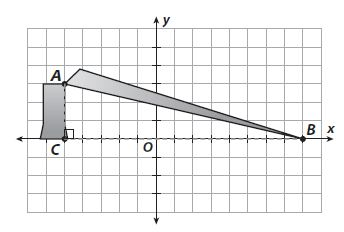a. What is the distance from A to B?
_______ meters

Explanation:
A= (-5,3)
B= (8,0)
Distance between A & B is
D= √{8-(-5)2 + (0-3)2
= √(13)2 + (-3)2
= √169+9
= √178
= 13.34  m.

Question 8.
b. What was the height of the tree before it fell?
_______ meters